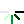Python3-内置函数和匿名函数## ☞一、内置函数

python一共为我们提供了68个内置函数。它们就是python提供给你直接可以拿来使用的所有函数。### ☞常用内置函数方法

1、print 输出

``````print('666',end='')
print('666')
print(1,2,3,4,5,sep='|')
666666
1|2|3|4|5
``````

2、input 输入

``````s = input("请输入内容 ： ")  #输入的内容赋值给s变量
print(s)  #输入什么打印什么。数据类型是str
``````

3、type(o) 返回变量o的数据类型

4、dir–查找对象的所有方法

dir() 默认查看全局空间内的属性，也接受一个参数，查看这个参数内的方法或变量

``````print(dir(list))  #查看列表的内置方法
print(dir(int))  #查看整数的内置方法
``````

5、locals()获取执行本方法所在命名空间内的局部变量的字典

globals()获取全局变量的字典

``````def func():
name = 'alex'
print(locals())
print(globals())
func()
``````

6、help（）----将你查询的对象所有信息都拿出来

help（list）–可以吧列表的所有用法信息都输出出来

7、abs（）----取绝对值

``````print(abs(-1))
print(abs(1))
def func(ret):
print(44)

ret = max(1,2,-3,key=abs)
print(ret)------   -3
#最小值
ret = min([1,2,3])
print(ret)------   1
#sum iterable,初始值
ret = sum([1,2,3],10)
print(ret)------   16
``````

8、callable---- 判断此变量是不是函数名

``````def func():pass
print(callable(func))  #参数是函数名，可调用，返回True
print(callable(123))   #参数是数字，不可调用，返回False
``````

9、id(o) o是参数，返回一个变量的内存地址

10、hash()-----通过哈希表的规则,将对象转换成哈希值

hash(o) o是参数，返回一个可hash变量的哈希值，不可hash的变量被hash之后会报错。

``````t = (1,2,3)
l = [1,2,3]
print(hash(t))  #可hash
print(hash(l))  #会报错
'''

TypeError: unhashable type: 'list'
'''
``````

hash函数会根据一个内部的算法对当前可hash变量进行处理，返回一个int数字。

*每一次执行程序，内容相同的变量hash值在这一次执行过程中不会发生改变。

11 、all（）----可迭代对象里面的所有的值转化成bool值如果都是True则,返回True

``````print(all([1,2,3,0]))
False
``````

12、十进制与二进制十六进制转化

``````十进制转化成二进制
print(bin(100))--------------0b1100100
#将十进制转化成八进制
print(oct(9))-----------------0o11
#将十进制转化成十六进制
print(hex(33))--------------0x21
``````

13、float（）----数据类型中浮点

``````print(1.35432,type(1.35432))
print(float(3))
1.35432
3.0
``````

14、enumerate (iterable,start 起始值) 枚举

``````l = ['手机','电话','充气娃娃',]
for i in enumerate(l,1):
print(i)
(1, '手机')
(2, '电话')
(3, '充气娃娃')
l = ['手机','电话','充气娃娃',]
for k,v in enumerate(l,1):
print(k,v)
1 手机
2 电话
3 充气娃娃
``````

15、eval（） 有返回值 除去字符串两边的引号,返回里面的内容

exec（） 没有返回值 除去字符串两边的引号,执行里面的代码

``````s = "{'name':'alex'}"
s1 = "1+2+3+4"
print(eval(s),type(eval(s)))
print(exec(s),type(exec(s)))
print(eval(s1),type(eval(s1)))
# {'name': 'alex'}
# None
# 10
``````
``````code = '''for i in range(10):
print(i)'''
print(exec(code))
0
1
2
3
4
5
6
7
8
9
None
``````

16、ord（） 输入字符返回Unicode对应的编码位置

``````print(ord('a'))
print(ord('b'))
print(ord('中'))
97
98
20013
``````

17、chr（）输入编码位置返回Unicode对应的字符

``````print(chr(97))
print(chr(20013))
a

``````

18、max（）里面可以加函数，以函数规则返回最大值

``````dic = {'k1':20,'k2':30,'k3':100}
def func(x):
return dic[x]
l1 = max(dic,key=func,)
print(l1)  #k3
dic = {3:20,2:30,1:100}
def func(x):
return x
l1 = max(dic,key=func,)
print(l1)  #3
``````

19、open() 打开一个文件，返回一个文件操作符(文件句柄)

20、`__import__`导入一个模块

``````import time
os = __import__('os')
print(os.path.abspath('.'))
``````

### ☞重点掌握

``````其他：input,print,type,hash,open,import,dir

str类型代码执行：eval,exec

``````

## ☞二、匿名函数

`````` #这段代码
def calc(n):
return n**n
print(calc(10))
#换成匿名函数
calc = lambda n:n**n
print(calc(10))
````````````函数名 = lambda 参数 ：返回值
#参数可以有多个，用逗号隔开
#匿名函数不管逻辑多复杂，只能写一行，且逻辑执行结束后的内容就是返回值
#返回值和正常的函数一样可以是任意数据类型
``````

目录